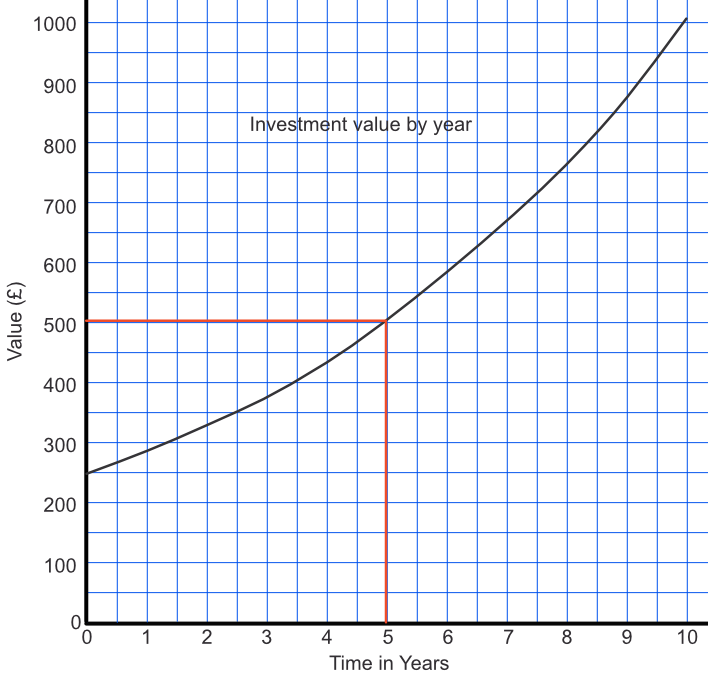Plot and Interpret Exponential Graphs

## Plot and Interpret Exponential Graphs

An exponential graph is generated from a function of the form f(x)=m^x.

The graph will be curved. They will typically show compound interest or compound growth. Because the graph is curved, use several points to plot the graph. In real-life situations, the graph normally shows positive values. In algebraic usage, the negative parts of a graph would also be shown.

## Example 1

£250 is invested with a compound rate of 15%. The table below shows how the saving grows over 10 years. Plot the graph, and determine what the investment is worth after 5 years.

 Time (years) 0 2 4 6 8 10 Value (£) 250 330 437 578 765 1011

After 5 years, £503 (any answer around £500 would be accepted). Draw up from 65 years to meet the graph, then along to read the value from the vertical scale.